# Proof for Close Packing of Congruent Identical Spheres

• A
fizixfan
I developed two algorithms for calculating the density of close packed congruent identical spheres in two different arrangements:
• A tetrahedron with four equilateral triangular faces, and
• A square pyramid with a square base and four equilateral triangular faces, as shown below.Figure 1. Tetrahedral ball stack.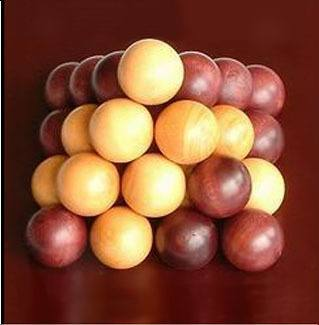Figure 2. Square pyramidal ball stack.

Here are the Excel-friendly algorithms (n = number of spheres along bottom row):

Density of Tetrahedral Stack (Dt):

Dt = (4*(2^0.5)*n*(n+1)*(n+2)*Pi())/(3*(2*n+2*3^0.5-2)^3 or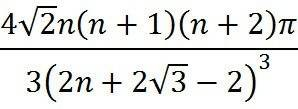(1)

Density of Square Pyramidal Stack (Dp):

Dp = ( n*(1+n)*(1+2*n)*Pi())/(3*((1+(2^0.5*n)))^3) or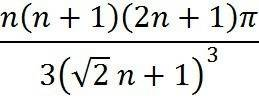(2)

I found that, as the number of spheres approaches infinity for both arrangements, that Dt = Dp = ≈ 0.74048... or π/√18.

I resorted to Wolfram Alpha with the following query: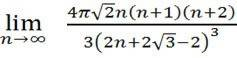=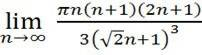(3)

and got the following result: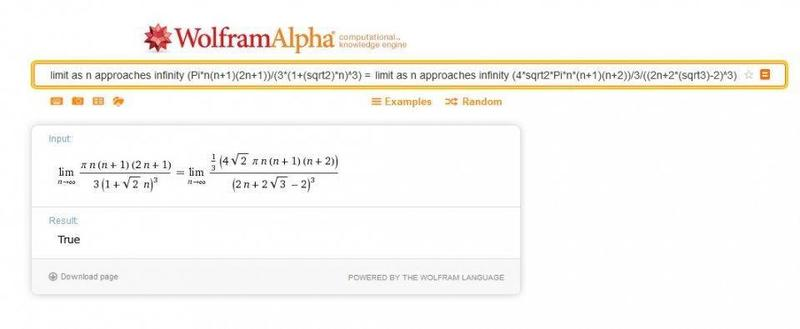(4)

True!

I then forwarded my calculations to Dr. Thomas C. Hales, who proved the Kepler Conjecture, asking him if Dt = Dp was correct, and he responded, saying,

"The reason for the equal densities in a tetrahedron and square pyramid is that they can both be viewed as part of the face-centered-cubic packing, each with a different set of exposed facets."

My question to you is this: can you provide a detailed proof that (1) = (2) as n→∞, i.e., that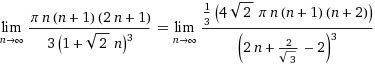is true?

Thanks for any input!

Fizixfan.

$$\frac{2 \pi n^3}{3 \cdot \sqrt{2}^3 n^3} = \frac{4 \pi \sqrt{2} n^3}{3 \cdot 2^3 \cdot n^3}$$
•